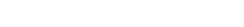﻿ Intervals## Intervals

An interval is the distance between two notes. There are two types of intervals, Harmonic, which are two notes played at the same time and Melodic which are two notes played separately. If the interval is greater than one octave then the interval can be described as Compound for example, C-E if it were a tenth could be described as a compound third.

NB. The terms used to describe intervals e.g. Melodic and Compound are not to be confused with the same words for the other subjects. A compound interval is completely different to compound time.

The interval is always counted from the bottom note upwards so if you had the notes C to A just below it, A would be the first note counting up to C making it a third.

All intervals from the tonic in the major key are described as being major with the exception of the fourth, fifth and octave which are perfect.

In the minor key the second degree is major, the third is minor, the fourth, fifth and octave are perfect and the sixth and seventh can be major or minor depending on whether the notes are raised.

There are certain rules for describing an interval that has been altered. If the interval is C to D, this would be a major 2nd. If the D were lowered a semitone, then the major 2nd would become a minor 2nd. If this were lowered another semitone (making D double flat), then the minor 2nd would become a diminished 2nd. Even though the notes would sound in unison, because they were written as C and D double flat, it is still a 2nd. If the interval C to D was raised a semitone then the interval would become an augmented 2nd. See the chart below for reference.